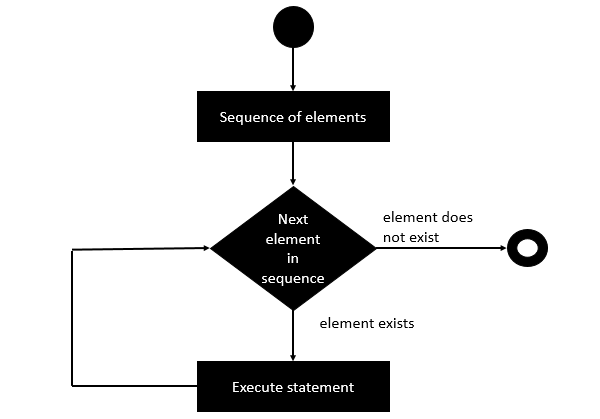# Clojure - Doseq Statement

The ‘doseq’ statement is similar to the ‘for each’ statement which is found in many other programming languages. The doseq statement is basically used to iterate over a sequence.

## Syntax

Following is the general syntax of the doseq statement.

```(doseq (sequence)
statement#1)
```

Following is the diagrammatic representation of this loop.## Example

Following is an example of the doseq statement.

```(ns clojure.examples.hello
(:gen-class))

;; This program displays Hello World
(defn Example []
(doseq [n [0 1 2]]
(println n)))
(Example)
```

In the above example, we are using the doseq statement to iterate through a sequence of values 0, 1, and 2 which is assigned to the variable n. For each iteration, we are just printing the value to the console.

## Output

The above code produces the following output.

```0
1
2
```
clojure_loops.htm